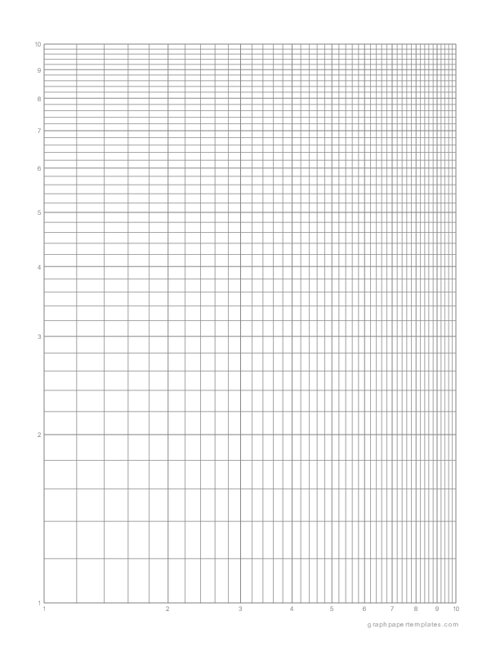# Log-Log Graph PaperPaper Size: Letter

Format: PDF

Size: 8.5" x 11"  -  ( 216 mm x 279 mm )

Layout: Portrait / Vertical

Color: Gray

Log-log graph paper is a type of graph paper that uses a logarithmic scale on both the x-axis and y-axis. This means that each unit on the axis represents a power of 10.

For example, on a log-log graph with an x-axis ranging from 1 to 10, the first unit would represent 10^1, or 10, while the second unit would represent 10^2, or 100.

If you are looking for linear scale on x-axis and logarithmic scale on y-axis, make sure to download log-log graph paper.

As a result, log-log graph paper is often used for plotting data that spans several orders of magnitude.

For instance, it can help track a population's growth over time or study the relationship between two variables that vary widely in value.

## Where is log-log graph paper used?

This versatile graph paper is commonly used in science and engineering projects.

• Log-log graph paper is specially designed for use with logarithmic scales. Both the x-axis and y-axis are logarithmically scaled, making it ideal for graphing logarithmic functions.
• This type of graph paper is often used in physics and engineering applications where logarithmic scales are needed.
• Log-log graph paper can also be useful for plotting data that spans several orders of magnitude.
• By using a logarithmic scale, it is possible to compress a large range of values into a more manageable space.

As a result, log-log graph paper can be a valuable tool for visualizing data sets that would otherwise be difficult to comprehend.

Log-Log Graph Paper

### You may also like:

1/2" Inch Graph Paper

Download free printable 1/2" inch graph paper with blue grid lines in portrait orientation. This type of graph paper has 1/2 inch squares, which makes it perfect for bullet journals, doodles and sketching.

Three Drop Brick Stitch Graph Paper

Free printable three drop brick stitch graph paper can be used to create a necklace, bracelet and earrings. Simply download, print and start beading.

Polar Graph Paper

Polar graph paper is a type of graph paper that is used to draw graphs and diagrams in polar coordinate system. This type of graph paper has radial lines and concentric circles instead of the usual horizontal and vertical lines.

Isometric Dot Paper

Isometric dot paper is a type of graph paper that uses dots instead of lines to create an isometric grid. This grid is used for drawing three-dimensional objects, such as cubes, pyramids, and spheres.

10 Squares Per Inch Graph Paper

10 squares per inch graph paper PDF printable has 10 squares per inch. It is perfect for graphing and drawing small diagrams. It can be used for a variety of purposes, from sketching and drawing to math and science.